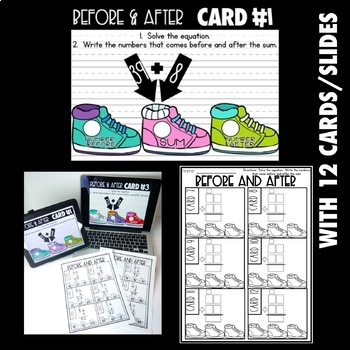2nd - 3rd
Subjects
Resource Type
Formats Included
• Zip
Pages
175 pages

Description

These TEN Addition with Regrouping Activities are very versatile! They can be used digitally through Power Point or Google Slides. They can also be printed with very little prep-just cut into fourths and done! They can be used as an independent activity, center, or scoot... so many options! Students do not need any extra materials, manipulatives, etc to complete the activities. All of the activities are for TWO DIGIT ADDITION WITH REGROUPING.

- Each activity comes with 12 questions: for digital version there are 12 slides, for printable version there are 12 cards. One activity has 8 cards/slides

- Each activity comes with a printable recording sheet. Students respond with their answers in a handwritten format.

- The directions for each activity are clearly stated on the student recording sheets.

- If you are using the printable cards, they can be given to a small group, used as a scoot, or placed in a math center.

- If you are using the digital version, students pull up the slides on Power Point or Google Slides (video directions included), they use the slide to answer their questions on their recording sheets.

Here are the Ten Activities included:

- Add and Compare: Students complete two addition with regrouping equations. Students compare the two sums.

- Choose and Add: There are 6 two digit numbers shown. Students choose two numbers to add together.

- Solve the Riddle: Students solve the equation. They will then use the key to find the letter needed to solve the riddle.

- True or False: Students have a problem shown. They will solve the problem and determine whether the sum shown is true or false.

- Number Bonds: Students are given the two addends. Students must find the sum

- Before and After: Students solve the equation. Students write the numbers that come before and after the sum.

- Find and Add: Students have two number lines shown with covered numbers. Students must first determine which numbers are missing. Then students add those two numbers together.

- Solve and Match: Students solve the equation. Then students find the emoji with the matching sum.

- Solve and Order: There are three problems shown. Students must solve each problem. Students order the sums from least to greatest.

- Make an Outfit: There are four numbers shown. Students choose two of the numbers based on the outfit they wish to make. Students add their outfit addends together.

Total Pages
175 pages
Not Included
Teaching Duration
N/A
Report this Resource to TpT
Reported resources will be reviewed by our team. Report this resource to let us know if this resource violates TpT’s content guidelines.